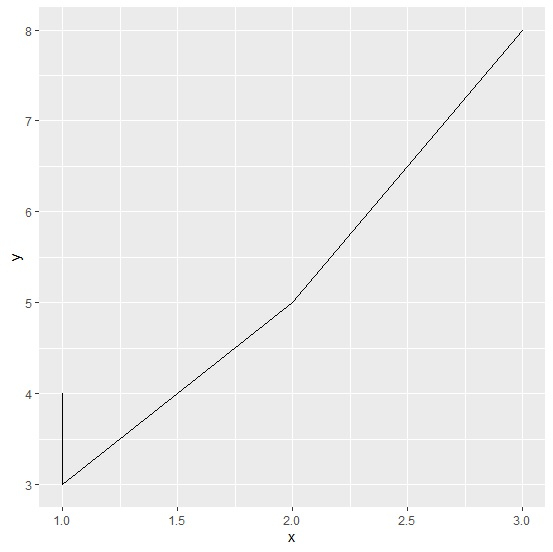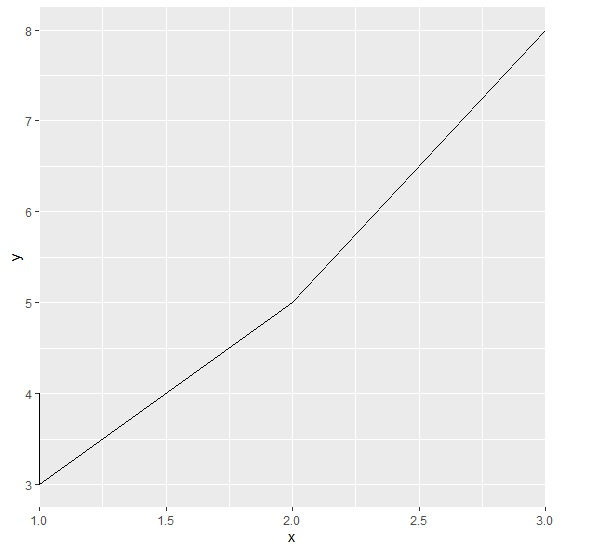# How to create a line chart using ggplot2 that touches the edge in R?

To create a line chart using ggplot2 that touches the edge we can follow the below steps −

• First of all, creating data frame.
• Then loading ggplot2 package and creating the line chart in default manner.
• After that creating the line chart with coord_cartesian function.

## Create the data frame

Let's create a data frame as shown below −

Live Demo

x<-rpois(5,2)
y<-rpois(5,5)
df<-data.frame(x,y)
df

On executing, the above script generates the below output(this output will vary on your system due to randomization) −

  x y
1 2 5
2 1 4
3 3 8
4 1 3
5 2 5

## Creating the line chart in default manner

Loading ggplot2 package and creating the line chart in default manner −

library(ggplot2)
x<-rpois(5,2)
y<-rpois(5,5)
df<-data.frame(x,y)
ggplot(df,aes(x,y))+geom_line()

### Output## Creating the line chart that touches the edges

Use coord_cartesian function of ggplot2 package to create the line chart where line touches the edges −

library(ggplot2)
x<-rpois(5,2)
y<-rpois(5,5)
df<-data.frame(x,y)
ggplot(df,aes(x,y))+geom_line()+coord_cartesian(xlim=c(1.09,2.91))

### Output